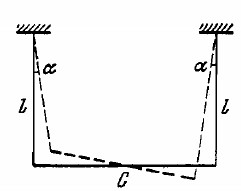A uniform rod of mass m = 1.5 kg suspended by two identical threads 1 = 90 cm in length (Fig) was turned through a small angle about the vertical axis passing through its middle point C. The threads deviated in the process through an angle a = 5.0°.Then the rod was released to start performing small oscillations.Find:
(a) the oscillation period;
(b) the rod's oscillation energy.Anonymous User Physics Oscillation 02 May, 2020 56 views